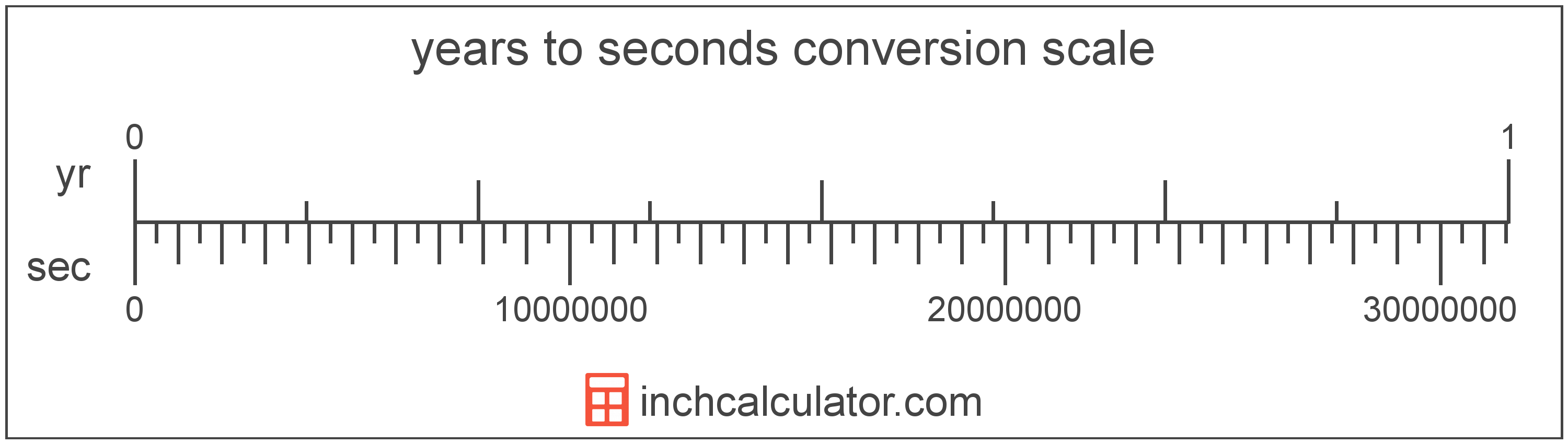# Seconds to Years Converter

Enter the time in seconds below to get the value converted to years.

Results in Years:1 s = 3.1689E-8 yr
Hint: use a scientific notation calculator to convert E notation to decimal

Do you want to convert years to seconds?

## How to Convert Seconds to Years

To convert a measurement in seconds to a measurement in years, divide the time by the following conversion ratio: 31,556,952 seconds/year.

Since one year is equal to 31,556,952 seconds, you can use this simple formula to convert:

years = seconds ÷ 31,556,952

The time in years is equal to the time in seconds divided by 31,556,952.

For example, here's how to convert 50,000,000 seconds to years using the formula above.
years = (50,000,000 s ÷ 31,556,952) = 1.584437 yr## What Is a Second?

The second has historically been defined as 1/60 of a minute or 1/86,400 of a day. According to the SI definition, the second is the duration of 9,192,631,770 oscillations of the frequency of light emitted from the atomic transition between the two hyperfine levels of the unperturbed ground state of the caesium 133 atom.

The second is the SI base unit for time in the metric system. Seconds can be abbreviated as s, and are also sometimes abbreviated as sec. For example, 1 second can be written as 1 s or 1 sec.

## What Is a Year?

One year is the time it takes for the Earth to complete its orbit around the Sun. There are 365 days in a year, with the exception of the leap year when there are 366.

We have leap years because the Earth actually takes 365.2425 days to revolve around the sun, but we round to 365 for convenience. However, since we miss a quarter of a day per revolution around the sun, this means that we add an extra day to the year once every 4 years, hence the leap year.

Years can be abbreviated as yr (plural yrs), and are also sometimes abbreviated as y. For example, 1 year can be written as 1 yr or 1 y, and 2 years can be written as 2 yrs.

## Second to Year Conversion Table

Table showing various second measurements converted to years.
Seconds Years
1 s 0.000000031689 yr
2 s 0.000000063377 yr
3 s 0.000000095066 yr
4 s 0.00000012675 yr
5 s 0.00000015844 yr
6 s 0.00000019013 yr
7 s 0.00000022182 yr
8 s 0.00000025351 yr
9 s 0.0000002852 yr
10 s 0.00000031689 yr
100 s 0.0000031689 yr
1,000 s 0.000031689 yr
10,000 s 0.000317 yr
100,000 s 0.003169 yr
1,000,000 s 0.031689 yr
10,000,000 s 0.316887 yr
100,000,000 s 3.1689 yr

## References

1. International Bureau of Weights and Measures, The International System of Units, 9th Edition, 2019, https://www.bipm.org/documents/20126/41483022/SI-Brochure-9-EN.pdf